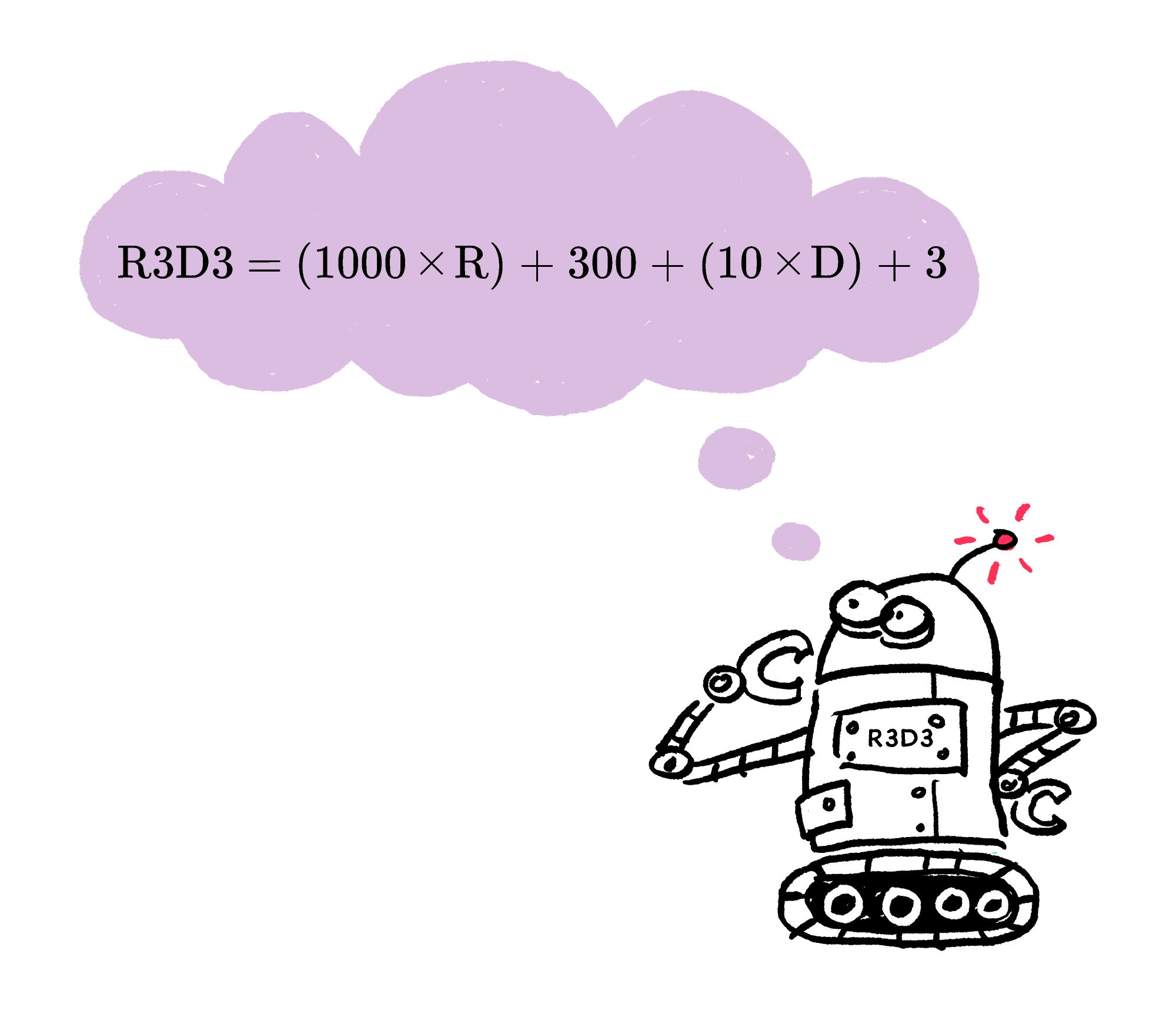### Mathematical Fundamentals

$\def\arraystretch{1.5} \begin{array} { c c c } & \large 1 & \large \color{#EC7300}{T} \\ + & \large \color{#EC7300}{T} & \large 6 \\ \hline & \large 7 & \large 1 \\ \end{array}$

Cryptograms are puzzles where capital letters stand in for the digits 1 to 9 of a number. If the same letter is used twice, it’s the same digit in both places.

Solve enough cryptograms, and you’ll start to see patterns — tricks that will help you solve puzzles like these faster and understand the mathematics more deeply.

# Reverse Engineering Arithmetic

$\def\arraystretch{1.5} \begin{array} { c c c } & \large 1 & \large \color{#EC7300}{T} \\ + & \large \color{#EC7300}{T} & \large 6 \\ \hline & \large 7 & \large 1 \\ \end{array}$

What digit in place of $\color{#EC7300}{T}$ would make this sum true?

# Reverse Engineering Arithmetic

$\def\arraystretch{1.5} \begin{array} {c c c c } & & \large \color{#D61F06}{A} & \large \color{#3D99F6}{B} \\ \large + & & \large \color{#D61F06}{A} &\large \color{#3D99F6}{B} \\ \hline & \large 1 & \large 3 & \large 8 \\ \end{array}$

What is the value of $\color{#D61F06}{A} \color{#333333}{?}$

# Reverse Engineering Arithmetic

$\def\arraystretch{1.5} \begin{array} {ccccc} \large& \large \color{#69047E}{Y} & \large \color{#69047E}{Y}\\ \large - & \large \color{#E81990}{Z}& \large \color{#E81990}{Z}\\ \hline \large & \large \color{#E81990}{Z} & \large 4 \end{array}$

If each letter represents a different nonzero digit, what must $\color{#69047E}{Y}$ be?

# Reverse Engineering Arithmetic

Practice works because, over time, you come up with a playbook of techniques that are frequently successful. But to get the most out of your practice, it can really help to intentionally dissect each of your solutions, extracting components of your strategies that might be reusable for future problems.In the previous three puzzles, did you use either of these two techniques? They'll both be very useful in the next two challenges.

• Converting all multi-digit numbers in the problem into algebraic expressions that take the place value of the letters into account. Here is an example:• Intentionally looking for places where it seems like one column of digits sums to a value greater than or equal to $10,$ causing an overflow (or 'carry') from one place value to the next.

# Reverse Engineering Arithmetic

$\def\arraystretch{1.5} \begin{array} {ccccc} \large& & \large \color{#EC7300}{E} & \large \color{#20A900}{G}\\ \large + & & \large \color{#EC7300}{E} & \large \color{#20A900}{G}\\ \hline \large & \large 1 & \large \color{#20A900}{G} & \large 0 \end{array}$

If each letter represents a different nonzero digit, what must $\color{#EC7300}{E}$ be?

# Reverse Engineering Arithmetic

$\large \begin{array} { l l l l } & & \color{#3D99F6}{Z} & \color{#3D99F6}{Z} \\ & & \color{#3D99F6}{Z} & \color{#3D99F6}{Z} \\ & & & \color{#3D99F6}{Z} \\ & & & \color{#3D99F6}{Z} \\ +& & &\color{#3D99F6}{Z} \\ \hline & 1 & 0 & 0 \end{array}$

What digit is ${\color{#3D99F6}{Z}}?$

# Reverse Engineering Arithmetic

×

Problem Loading...

Note Loading...

Set Loading...